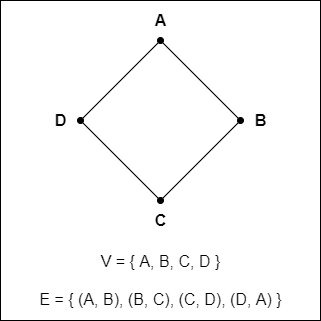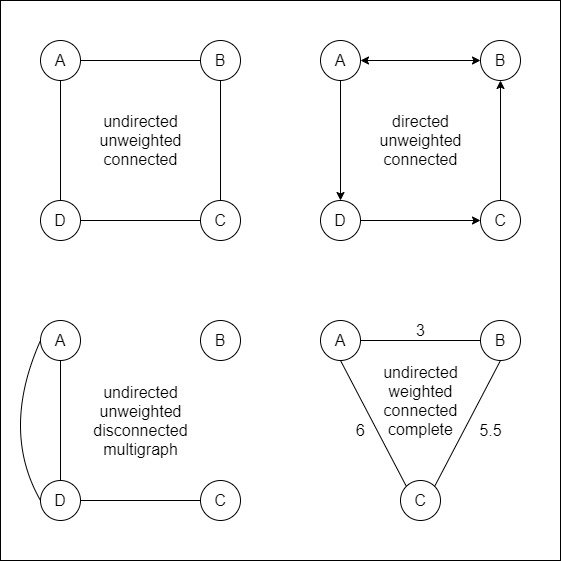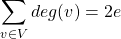# Mathematics - Discrete Mathematics - Graphs

in STEMGeekslast year[Image 1]

## Introduction

Hey it's a me again @drifter1!

Today we continue with Mathematics, and more specifically the branch of "Discrete Mathematics", in order to get into Graphs.

So, without further ado, let's get straight into it!

## Graph Theory

Graph Theory is the branch of mathematics concerned with the study of objects known as graphs. Graphs consist of vertices (or nodes) which are connected by edges.

Many problems can be represented and solved using them, and thus Graph theory finds applications in not only mathematics, but also physics and computer science as well.

## Graphs

A graph is defined as G = (V, E), where:

• V is the set of vertices or points or nodes
• E is the set of ordered pairs of distinct vertices known as edges

For example:Let's get through some terminology now...

### Vertices

Two distinct vertices u and v of a graph are adjacent if an edge e = (u, v) connects them. The set of all vertices adjacent to a given vertex v is defined as N(v) and known as the neighborhood of v. The number of edges that are incident to a given vertex v is defined as the degree of that vertex and denoted as deg(v).

Vertices of degree one are called pendant. In a similar manner, vertices with odd degree are odd, and vertices with even degree are even.

In the example graph mentioned previously each vertex is of degree 2, and so all vertices are of even degree.

### Types

Graphs can be directed or undirected. In a directed graph each edge is assigned a direction, and so the edge or ordered pair (u, v) starts from u and ends at v.

When multiple edges connect the same set of vertices then the graph is known as a multigraph.

When its possible to traverse from any vertex to any other vertex then the graph is connected, otherwise it is disconnected. Additionally, in the special case where all vertices are connected by edges with each other that graph is considered complete.

A graph which consists only of isolated vertices, and no edges, is known as a null graph.

In some cases graphs can also be weighted. In a weighted graph each edge is assigned a positive number w called weight which specifies the "cost" of traversing through them. Graphs with no weights are unweighted.

For example:### Handshake Lemma

For any undirected graph G = (V, E):where e is the number of edges.

Based on that theorem, for any graph the number of vertices with odd degree must be even.

## RESOURCES:

### Images

Block diagrams and other visualizations were made using draw.io.

## Final words | Next up

And this is actually it for today's post!

Next time we will continue on with more on Graphs...

See ya!Keep on drifting!

Posted with STEMGeeks

Sort:#### You have received a 1UP from @luizeba!

The following @oneup-cartel family members will soon upvote your post:
`@stem-curator`
And they will bring !PIZZA 🍕